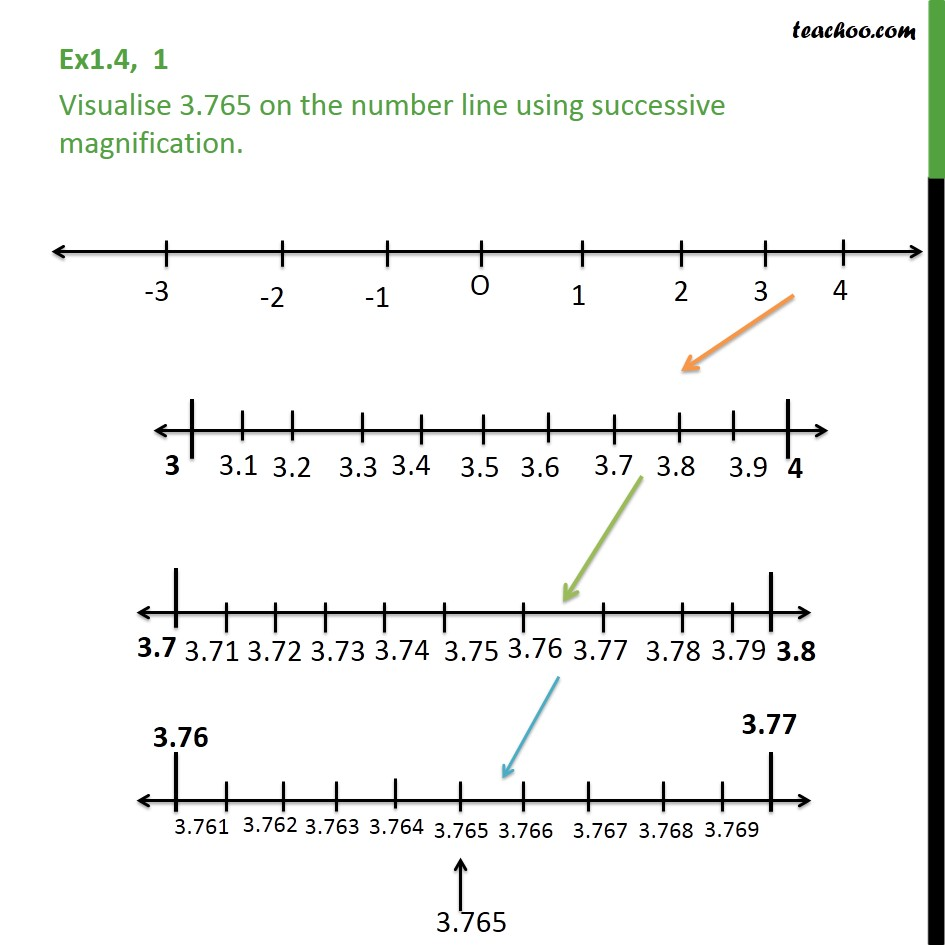Learn All Concepts of Chapter 1 Class 9 - FREE. Check - Chapter 1 Class 9 Maths1. Chapter 1 Class 9 Number Systems
2. Concept wise
3. Real numbers on a number line

Transcript

Ex 1.4, 1 Visualise 3.765 on the number line using successive magnification. Here, we follw these steps. 1. We draw number line from 3 to 4 with markings as 3.1, 3.2, 3.3, .... 2. Then we draw number line from 3.7 to 3.8, with markings as 3.71, 3.72, 3.73, 3.74, 3.75, .... 3. Then we draw number line from 3.76 to 3.77, with markings as 3.761, 3.762, 3.763, 3.764, 3.765, .... And we can now mark 3.765

Real numbers on a number line